Home > CC4 > Chapter A > Lesson A.1.1 > ProblemA-9

A-9.
1. The word “evaluate” has many different meanings. In math, when you are asked to evaluate an expression for a specified value of the variable(s), you are being asked to determine the value of the expression. You do this by replacing a variable with the given number and calculating the result. Homework Help ✎

2. Evaluate the expressions below for the given values.

1. −4d + 3 if d = −1

2. km if k = 4 and m = −10

3.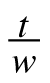if t = 6 and w = −3

4. x2 + y2 if x = 7 and y = 5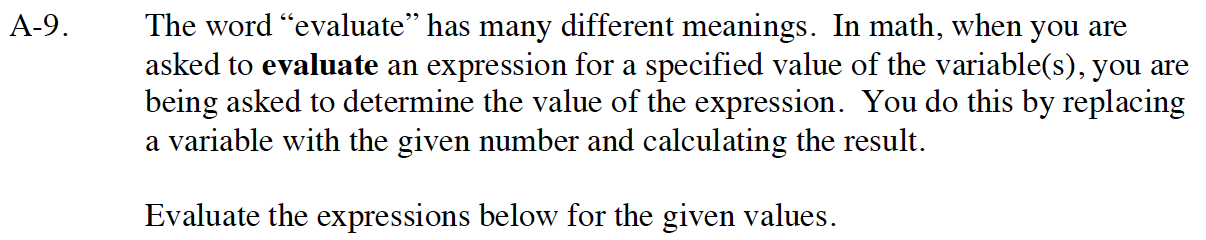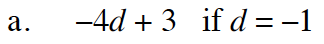Substitute −1 for d.

−4(−1) + 3

Simplify the terms and combine them.

4 + 3 = 7

7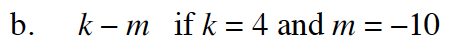Follow the steps in part (a).

Put everything you substituted inside parentheses so you do not lose your negative signs.

(4) − (−10)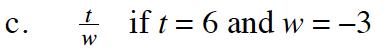Follow the steps in part (a).

−2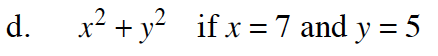Follow the steps in part (a).